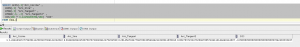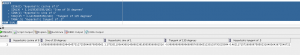# PL/SQL – Number Functions

Hi Folks,

Functions are similar to operators in that they manipulate data items and return a result. Functions differ from operators in the format of their arguments. This format enables them to operate on zero, one, two, or more arguments. SQL functions are built into Oracle Database and are available for use in various appropriate SQL statements.

Numeric functions accept numeric input and return numeric values. Most numeric functions that return NUMBER values that are accurate to 38 decimal digits. The transcendental functions COS, COSH, EXP, LN, LOG, SIN, SINH, SQRT, TAN, and TANH are accurate to 36 decimal digits. The transcendental functions ACOS, ASIN, ATAN, and ATAN2 are accurate to 30 decimal digits.

## Most Used Functions

 SYNTAX DESCRIPTION ABS(n) ABS returns the absolute value of n. CEIL(n) CEIL returns smallest integer greater than or equal to n. EXP(n) EXP returns e raised to the nth power, where e = 2.71828183 FLOOR(n) FLOOR returns largest integer equal to or less than n. LN(n) LN returns the natural logarithm of n, where n is greater than 0. LOG(n2, n1) LOG returns the logarithm, base n2, of n1 MOD(n2, n1) MOD returns the remainder of n2 divided by n1. Returns n2 if n1 is 0. POWER(n2, n1) POWER returns n2 raised to the n1 power. REMAINDER(n2, n1) REMAINDER returns the remainder of n2 divided by n1. ROUND(n, interger) ROUND returns n rounded to integer places to the right of the decimal point. If you omit integer, then n is rounded to 0 places. SIGN(n) SIGN returns -1 if n<0, 0 if n=0, and 1 if n>0 SQRT(n) SQRT returns the square root of n. TRUNC(n1, n2) The TRUNC (number) function returns n1 truncated to n2 decimal places. If n2 is omitted, then n1 is truncated to 0 places.
```SELECT
ABS(-15) "Absolute"
, CEIL(268651.8) "CEIL"
, EXP(4) "e to the 4th power"
, FLOOR(15.7) "Floor"
, LN(95) "Natural log of 95"
, LOG(10,100) "Log base 10 of 100"
, MOD(11,4) "Modulus"
, POWER(3,2) "Raised"
, REMAINDER(21.23, 1) "Remainder"
, ROUND(15.193,1) "Round"
, SIGN(-15) "Sign"
, SQRT(25) "Square root"
, TRUNC(15.79,1) "Truncate"
FROM DUAL;```## Trigonometric Functions

 SYNTAX DESCRIPTION ACOS(n) ACOS returns the arc cosine of n. ASIN(n) ASIN returns the arc sine of n. ATAN(n) ATAN returns the arc tangent of n. ATAN(n1, n2) ATAN2 returns the arc tangent of n1 and n2. COS(n) COS returns the cosine of n (an angle expressed in radians). COSH(n) COSH returns the hyperbolic cosine of n. SIN(n) SIN returns the sine of n (an angle expressed in radians). SINH(n) SINH returns the hyperbolic sine of n. TAN(n) TAN returns the tangent of n (an angle expressed in radians). TANH(n) TANH returns the hyperbolic tangent of n.
```SELECT
ACOS(.3)"Arc_Cosine"
, ASIN(.3) "Arc_Sine"
, ATAN(.3) "Arc_Tangent"
, ATAN2(.3, .2) "Arc_Tangent2"
, COS(180 * 3.14159265359/180) "COS"
FROM DUAL;``````SELECT
COSH(0) "Hyperbolic cosine of 0"
, SIN(30 * 3.14159265359/180) "Sine of 30 degrees"
, SINH(1) "Hyperbolic sine of 1"
, TAN(135 * 3.14159265359/180)  "Tangent of 135 degrees"
, TANH(.5) "Hyperbolic tangent of .5"
FROM DUAL;```